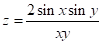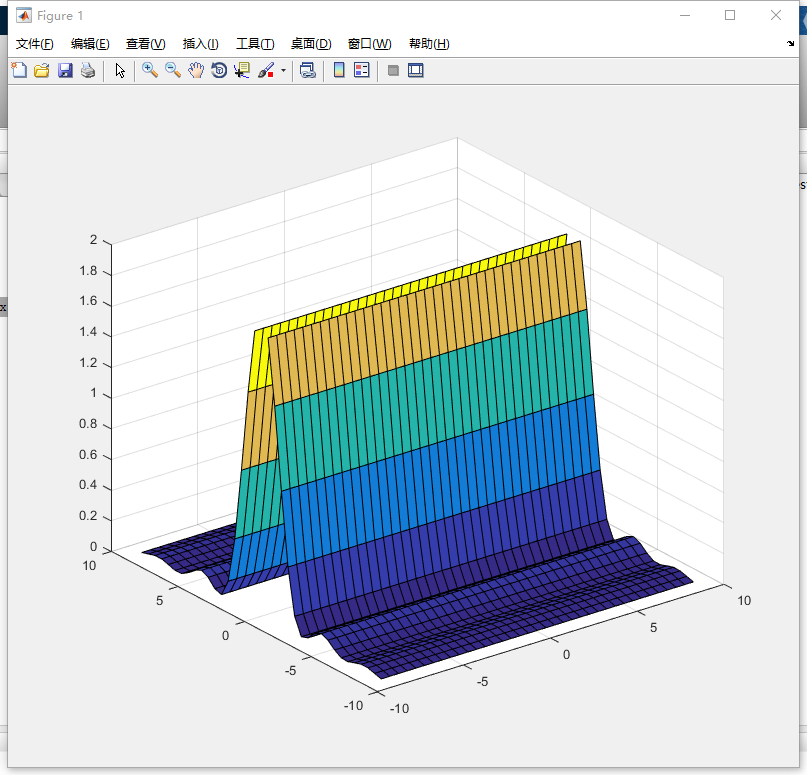hokutosei-019 2022-04-10 23:46 采纳率: 75%

# MATLAB，二元函数问题

1. 编写一段matlab程序，绘制出二元函数三维网线图，要求如下：
（1）X ，Y 的取值范围为-9<=X <=9，-9<=Y <=9 ；
（2） X， Y每隔0.5取一个点；
（3）图形的线型和颜色由MATLAB自动设定
• 写回答

#### 1条回答默认 最新

•A-Chin 2022-04-11 00:57
关注
``````x = -9.0 : 0.5 : 9.0;
y = -9.0 : 0.5 : 9.0;
z = zeros(length(x), length(y));
for i = 1 : length(x)
for j = 1 : length(x)
z(i, j) = 2 * sin(x(i)) * sin(y(i)) / (x(i) * y(i));
end
end

figure;
surf(x,y,z);
``````本回答被题主选为最佳回答 , 对您是否有帮助呢?
评论

#### 悬赏问题

• ¥15 关于非编程寻迹小车的分析
• ¥15 java导出EXCEL这里已经执行成功了，但是流浪器没有下载
• ¥15 帮我把代码改的能正常运行就行
• ¥50 有限元修正、模型修正、最小二乘法，详细步骤
• ¥15 用plc编写梯形程序
• ¥15 关于#物联网#的问题：新大陆AIOT中，按照手册教程进行添加，设置完成后网关一直不上线，显示Never这是网络服务的信息
• ¥15 这个要用一维热方程但是我不知道怎么运用这个公式
• ¥15 OpenFOAM多孔介质传热模型建模
• ¥15 QT 实现 RSTP 语音对讲功能
• ¥15 用C语言写的一个程序遇到了两个问题第一是偏移正确但读取不到坐标，第二个问题是自己定义的函数实现不了获取指定进程模块。